• 论文研究-半参数STAR模型的估计应用.pdf, 文中首次提出了一个新的STAR模型，在保留了转换函数的前提下，让转换变量以非参数的形式进入转换函数，从而有效减少了模型误...
• 极大似然估计贝叶斯估计是参数估计中的一种方法，以贝叶斯思想为基础，而贝叶斯思想在机器学习中经常用到。机器学习中常涉及贝叶斯网络，最终的问题都是转化为参数求解。贝叶斯参数估计是这些问题的基础版本。前方...
文章目录极大似然估计贝叶斯估计（Bayes Estimation）损失函数风险函数贝叶斯风险如何最小化贝叶斯风险（贝叶斯估计）结论三种常用损失函数的贝叶斯估计计算Square ErrorAbsolute ErrorUniform Error如何确定先验分布（先验知识）与后验分布共轭先验（Conjugate Prior）后验分布的计算泊松分布的例子二项分布的例子（点击率的贝叶斯平滑）二项分布贝叶斯估计的应用示例（点击率的贝叶斯平滑）
极大似然估计
贝叶斯估计是参数估计中的一种方法，以贝叶斯思想为基础，而贝叶斯思想在机器学习中经常用到。机器学习中常涉及贝叶斯网络，最终的问题都是转化为参数求解。贝叶斯参数估计是这些问题的基础版本。前方高能预警，本文的讲解比较理论。
实际问题中我们会有很多数据，比如一篇文章中每个单词的词频等。我们得到的数据通常用$X$表示，也称为样本。我们还会假设这些数据服从某一个分布，例如最常用的正态分布，这时可以将问题表示为$X \sim N(\mu, \sigma)$，$\mu$和$\sigma$表示正态分布的两个参数。如果这两个参数已知，这个分布就确定了，从而可以知道数据$X$的许多性质。这种情况下，参数估计就是要估计$\mu$和$sigma$的值。最常用的参数估计方法是极大似然（或最大似然估计）估计。
一般的最大似然法求解两个参数的基本步骤是：

假设每个样本$X_i$是独立同分布（iid）的，即每一个样本都有$X_i \sim N(\mu, \sigma)$.
求所有样本$X$的联合分布
因为是iid，所以$X$的联合分布等于每个样本$X_i$的概率密度函数的乘积，即：
$L(\mu, \sigma^2; \boldsymbol{x}) = f(\boldsymbol{x}) = \left(\frac{1}{\sqrt{2\pi\sigma^2}}\right)^n \exp\left\{-\sum_{i=1}^n \frac{(x_i - \mu)^2}{2\sigma^2}\right\}$
对上述联合分布的概率密度函数取对数，即：
$\ell(\mu, \sigma^2; \boldsymbol{x}) = \log L(\mu, \sigma^2; \boldsymbol{x}) = -\frac{n}{2}\log\left(2\pi\sigma^2\right) -\sum_{i=1}^n \frac{(x_i - \mu)^2}{2\sigma^2}$
对上述函数分别求$\frac{\partial \ell}{\partial \mu}$和$\frac{\partial \ell}{\partial \sigma^2}$，并令它们等于0，进而求得极值
分别对$\mu$和$\sigma^2$求二阶偏导，验证极值是最大值

上述步骤是极大似然法的求解步骤，用到的信息都是已知样本的信息。但是通常在估计参数时我们可能已经对参数有了一个大概的了解，比如已经知道$\mu$和$\sigma^2$的取值范围。仅仅知道取值范围还太简单，有时会更进一步假设$\mu$和$\sigma^2$的取值服从某个分布，这样问题就变成了在正态分布中，要估计期望$\mu$和方差$\sigma^2$的值，但与极大似然法不同的是，我们事先已经知道了$\mu$和$\sigma^2$的取值是服从某种分布的，这个信息如果不用到参数估计中有点浪费了，于是问题变成：如何将这两个参数的分布结合到参数估计过程中？ 贝叶斯估计解决了这个问题。
贝叶斯估计（Bayes Estimation）
上述提到的在估计参数之前对参数已经有了了解称为参数的先验知识。贝叶斯估计即在估计过程中将先验知识也考虑了进去，博众家之长总是好的。先验知识可以是一个具体的值，也可以是取值范围，也可以是一个函数（某种分布）。实际应用中，通常会将参数的先验知识视作一个分布，那么这个参数就会有一个概率密度函数（pdf），这个pdf叫做待估计参数的先验概率。
一般待估计的一维参数用$\theta$表示，多维用粗体$\boldsymbol{\theta}$. 先验概率密度函数用符号$\pi(\theta)$表示。样本的概率密度函数用$f(x|\theta)$表示，其中加入$\theta$是表示该pdf跟$\theta$有关，同时说明要估计的参数是$\theta$.
参数估计必然会涉及到『损失』的概念，用于表示参数的估计值与真实值之间的差距。差距越小，损失越小，说明参数估计得约准确。
贝叶斯估计涉及到三个损失概念，他们长的很像：

损失函数（Loss Funcition）
风险函数（Risk Function）
贝叶斯风险（Bayes Risk）

贝叶斯估计的目的是：结合参数的先验知识，使得参数的估计值令贝叶斯风险达到最小。简单说就是最小化贝叶斯风险。
下面解释这三个概念。
损失函数
在参数估计问题中，评价估计的好坏就是看估计出来的参数与真值的差距有多小。估计出来的参数通常用$\hat{\theta}$表示，参数的真值用$\theta$表示。$\theta$与$\hat{\theta}$的差距就是损失函数。
损失函数有好几种定义方式，常见的如下：

$L(\hat{\theta}, \theta) = (\hat{\theta} - \theta)^2$
$L(\hat{\theta}, \theta) = |\hat{\theta} - \theta|$
\begin{aligned} L(\hat{\theta}, \theta) = \begin{cases} 0 &\text{if |\theta - \hat{\theta}| \leqslant \Delta} \\ 1 &\text{if |\theta - \hat{\theta}| > \Delta} \end{cases} \end{aligned}

当估计值与真实值无限接近时，损失函数都会无限接近0，相当于没有损失。损失函数中的估计值$\hat{\theta}$是通过样本计算出来的。比如正态分布中的$\mu$，我们可以用样本均值来估计$\mu$（即将样本均值当成$\mu$），即$\hat{\mu} = \frac{1}{n}\sum^n X_i = \bar{\boldsymbol{X}}$。类似地，也常用样本方差来估计$\sigma^2$. 但是注意到$\hat{\mu}$和$\hat{\sigma}^2$的值都跟样本的个数有关，即都跟$n$有关。如果$\frac{1}{n}\sum_{i=0}^n X_i$是$\mu$的估计值，那$\frac{1}{n-1}\sum_{i=0}^{n-1} X_i$是$\mu$的估计值吗？那$\frac{1}{n-6}\sum_{i=0}^{n-6} X_i$呢？可以看到$n$不一样，估计值也不一样，到底用哪个$n$（用多少个样本）来计算损失函数呢？这时容易（其实不容易）想到，既然损失函数可以因为$\hat{\theta}$的不同而有不同的值，那就求平均。通过判断损失的平均值的大小来判断参数估计得好不好。求平均是很自然的想法，但平均通常是相对样本来说的，如果是总体，我们通常说期望。这时就要引入风险函数了。
风险函数
风险就是损失函数关于$\hat{\theta}$的期望。
既然估计值$\hat{\theta}$是随$n$的变化而变化的，那也可以将$\hat{\theta}$视为随机变量，进而可以计算损失函数的期望。于是风险函数的定义如下：
$R(\hat{\theta}, \theta) = E_{\hat{\theta}} \left[L(\hat{\theta}, \theta) \right]$
$E_{\hat{\theta}}$表示对$\hat{\theta}$求期望（注意真值$\mu$是固定的，不是变量）。我们的目标是：求出一个$\hat{\theta}$，使得风险最小（最小化风险）。
例如，要估计期望$\mu$，假设损失函数是$L(\hat{\mu}, \mu) = (\hat{\mu} - \mu)^2$，如果用样本均值作为$\mu$的估计值（即$\hat{\mu} = \frac{1}{n}\sum_{i=0}^n X_i$），这样的风险是多少？
$E \left[L(\hat{\mu}, \mu) \right] = E(\bar{\boldsymbol{X}} - \mu)^2 = E\bar{\boldsymbol{X}}^2 - 2\mu E\bar{\boldsymbol{X}} + \mu^2 \ \$
算出上式中的$E\bar{\boldsymbol{X}}^2$和$E\bar{\boldsymbol{X}}$就可以知道风险是多少。其实上式的风险是0，已经是最小值了。这个结果说明当使用样本均值来估计总体期望时，风险最低，所以一般都用样本均值来代替总体期望。
到目前为止并没有用到前面提过的先验知识$\pi(\theta)$，仅仅定义了损失函数和风险函数。上式的风险函数求出了一个具体的值，但很多情况没有那么简单，求出的风险是一个函数表达式，而不是值。如果求出来的风险是一个值，其实可以不用贝叶斯估计（上面也一直没有用到$\theta$的任何先验知识）。贝叶斯估计通常用于风险不可以直接比较的情况。
这时就要引入贝叶斯风险的概念了。贝叶斯风险中就用到了$\theta$的先验分布$\pi(\theta)$.
贝叶斯风险
注意到风险函数$R(\hat{\theta}, \theta)$是$\hat{\theta}$的函数，前面也提到真值$\theta$是一个固定的值，不是变量。但是，我们会在估计$\theta$之前知道一些$\theta$的知识，比如说$\theta$的分布$\pi(\theta)$（这个分布是怎么知道的呢？下文有讲解）。既然$\theta$是有一个概率分布的，那么此时$\theta$也变成一个随机变量了，所以 $R(\hat{\theta}, \theta)$同时是$\hat{\theta}$和$\theta$的函数。 这时要怎么使用$\pi(\theta)$呢？下面定义贝叶斯风险。
贝叶斯风险：
$Bayes\ Risk = E_{\theta} \left[R(\theta, \hat{\theta})\right] = \int R(\theta, \hat{\theta})\pi(\theta) d\theta \text{\ (期望的定义)}$
上式表示：贝叶斯风险是风险函数在$\theta$上的期望。
回顾一下期望的定义。如果随机变量用$\boldsymbol{X}$表示，其概率密度函数是$f(\boldsymbol{x})$，那么$\boldsymbol{X}$的期望$E\boldsymbol{X} = \int xf(x)dx$. 对照期望的积分形式，可以看到$\pi(\theta)$就是$\theta$的概率密度函数，随机变量是$\theta$. $R(\theta, \hat{\theta})$是关于随机变量$\theta$的一个函数，所以Bayes Risk就是在求风险函数的期望。总的来说：风险函数是损失函数关于$\hat{\theta}$的期望，而贝叶斯风险是风险函数关于$\theta$的期望。 所以贝叶斯风险是一个双期望。更进一步，$\hat{\theta}$是关于随机变量$X$的函数（$\hat{\theta}$总是通过样本$\boldsymbol{X}$求出来），所以也可以说：风险函数是损失函数关于$\boldsymbol{x}$的期望。这样就将风险与$\theta$的先验知识关联起来了。
之所以叫贝叶斯风险是因为引入了一个先验分布$\pi(\theta)$. 『先验』这个词本身就是贝叶斯理论的一部分。
如何最小化贝叶斯风险（贝叶斯估计）
终于到达贝叶斯估计的核心了，即如何找到一个$\hat{\theta}$，使得贝叶斯风险最小。问题转化为求$\int R(\theta, \hat{\theta})\pi(\theta) d\theta$的最小值。
这是一个积分形式的函数，如果知道$R(\theta, \hat{\theta})$和$\pi(\theta)$的具体形式可以直接求出最小值。不过如果仅仅根据这个抽象的形式，也可以求出最小值的形式。
下面给出最小化贝叶斯风险的推导过程，不想看推导过程可以直接看最后结论。
为了跟一些书的符号统一，下面引入一个新的符号：$a = \hat{\theta}$. 即用字母$a$来表示参数的估计值$\hat{\theta}$.
字母$a$表示action，表示采取某一动作求得$\theta$的估计。在参数估计问题中，$a$就是$\hat{\theta}$. 所以$R(\theta, \hat{\theta}) = R(\theta, a)$
因为$R(\theta, a)$的本质也是求期望，先将其转换为积分的形式。
$R(\theta, a) = E_a\left[L(\theta, a)\right] = \int L(\theta, a(x))f(x|\theta) dx$
因为$a$是$x$的函数，所以对$a$求期望就是在$x$上求期望，进而转换为对$x$求积分。同时$x$的概率密度函数是已知的，记为$f(x|\theta)$，表示$x$的pdf跟$\theta$有关，也表示条件概率密度函数。
既然在$\theta$给定的条件下，$x$的条件pdf是$f(x|\theta)$（此时已经将$\theta$当成随机变量看待了），而且$\theta$的分布又是$\pi(\theta)$，那么根据条件概率的定义，可以求出$x$的边缘概率密度函数：
$f(x) = \int f(x|\theta) \pi(\theta)\ d\theta \ \ \text{(边缘概率定义)}$
所以有：
\begin{aligned} \int R(\theta, a)\pi(\theta) d\theta &= \int_{\theta} \left[\int_x L(\theta, a(x))\ f(x|\theta)\ dx\right] \pi(\theta)\ d\theta & \text{(带入上式展开)}\\ &= \int_{\theta} \int_x L(\theta, a(x)) \frac{f(x|\theta) \pi(\theta)}{f(x)} f(x)\ dx\ d\theta & \ \text{(除以一个f(x)再乘以一个f(x)结果不变)} \\ &= \int_{\theta} \int_x L(\theta, a(x)) \pi(\theta|x)\ f(x)\ dx\ d\theta & \ \text{(贝叶斯定理)} \\ &= \int_x \left[\int_{\theta} L(\theta, a(x)) \pi(\theta|x)\ d\theta \right] f(x)\ dx\ & \ \text{(交换积分顺序)} \\ \end{aligned}
最后一步无法化简了，那就分析上式中最后一项。
通过贝叶斯定理，求出了$\pi(\theta|x)$. 观察最后一项中括号中的式子，$\pi(\theta|x)$是一个pdf，中括号中的这一项很像期望的定义。实际上中括号这一项就是在$\pi(\theta|x)$上求损失函数$L(\theta, a(x))$的期望。
回顾贝叶斯估计的目的：求出$\hat{\theta}$（在这里是$a(x)$），使得贝叶斯风险最小（即$\int R(\theta, a)\pi(\theta) d\theta$最小）。
中括号中的那一项是对$\theta$积分，积分后不会有$\theta$，从而整个式子只剩下$x$. 而回想一下贝叶斯估计的目的，$x$并不是我们要关心的。所以贝叶斯估计就是要计算中括号一项，使得中括号内的积分最小，最终还是回到了损失函数上。
上面提到中括号一项看起来像是期望的定义，其实这一项称为posterior expected risk. 记作：
$\int_{\theta} L(\theta, a(x)) \pi(\theta|x)\ d\theta = E_{\pi} L(\theta, a(x))$
$E_{\pi}$表示在$\pi(\theta|x)$上求期望。$\pi(\theta|x)$就叫做 $\theta$的后验分布，即在知道数据$x$后$\theta$的分布。所以贝叶斯估计就是：求$\hat{\theta}$，使得损失函数在$\theta$的后验分布上的期望最小。
此时可以发现，$损失函数L(\theta, a(x))$是自己设计的，比如前面提到的那三种。如果知道$\pi(\theta)$，根据贝叶斯定理，容易求出$\pi(\theta|x)$；而$\pi(\theta)$也是我们自己定义的（先验知识，肯定是事先就知道了的，不知道也可以假设），所以这个posterior expected risk不难求得。至于如何计算后验分布，后面有讲解。
结论
贝叶斯参数估计的步骤：

拿到数据，知道数据的分布（或者定义数据的分布），记为$f(\boldsymbol{x}|\theta)$，要估计的参数记为$\theta$
定义损失函数$L(\theta, \hat{\theta})$
定义（或者假设）$\theta$的先验知识或先验分布$\pi(\theta)$
根据贝叶斯定理求出后验分布$\pi(\theta|\boldsymbol{x}) = \frac{f(\boldsymbol{x}|\theta)\pi(\theta)}{f(\boldsymbol{x})}$
最小化如下式子：
$\arg \min \int L(\theta, \hat{\theta}) \pi(\theta|\boldsymbol{x})\ d\theta = \arg \min E_{\pi} L(\theta, \hat{\theta})$

上面最后一步，涉及积分以及最小值求解，看起来十分麻烦。所幸，在特定的损失函数形式下，上面最后一步可以化简，并不需要完全用到积分。下面讲解在三种特定损失函数形式下贝叶斯估计的计算方法。
理论总是简单的，上述的求解过程后面附有例子，可以温习一下。
三种常用损失函数的贝叶斯估计计算
Square Error
square error就是
$L(\theta, a) = (\theta - a)^2$
下面这直接给出结论：
若损失函数是square error，那么当$a$等于$\theta$在$\pi(\theta|x)$上的期望时，贝叶斯风险最小。
上述结论证明涉及到其它知识，略过。
上述结论说明，如果知道$\pi(\theta|x)$的形式，那么只需求$\int\theta\pi(\theta|x)\ d\theta$就可以了，实际上就是期望。例如，如果$\pi(\theta|x)$是正态分布，$\pi(\theta|x) \sim N(\eta, \tau)$，那么$a = \eta$，$\eta$就是参数$\theta$的估计。
Absolute Error
Absolute error定义如下：
$L(\hat{\theta}, \theta) = |\hat{\theta} - \theta|$
下面直接给出结论：
若损失函数是square error，那么当$a$等于数据$X$的中位数时，贝叶斯风险最小。
这个结论说明：如果使用square error作为损失函数，不需要定义$\theta$的后验分布，直接取中位数。
Uniform Error
uniform error的形式为：
\begin{aligned} L(\hat{\theta}, \theta) = \begin{cases} 0 &\text{if |\theta - \hat{\theta}| \leqslant \Delta} \\ 1 &\text{if |\theta - \hat{\theta}| > \Delta} \end{cases} \end{aligned}
下面直接给出结论：
若损失函数是uniform error且$\Delta$很小，当$a = \arg \max \pi(\theta|x)$时，即$a$等于$\theta$后验分布的最大值时，贝叶斯风险最小。
上述结论说明，在uniform error的情况下，如果知道$\pi(\theta)$的形式，那么求它的最大值即可。例如，如果$\pi(\theta|x)$是正态分布，$\pi(\theta|x) \sim N(\eta, \tau)$，正态分布的最大值在均值处取得，所以$\theta$的估计值为$\eta$，与square error一样。
如何确定先验分布（先验知识）与后验分布
前面一直提到先验知识或者先验分布，偶尔混用。这是因为$\theta$的先验知识$\pi(\theta)$可以有很多种形式，可以是一个数，可以是离散的几个数，也可以是个概率分布函数，此时称为后验分布。
但是对于后验分布，它只能是一个概率分布形式，即$\pi(\theta|x)$必须满足概率密度函数的定义，而$\pi(\theta)$却不一定。原因是最后求贝叶斯风险最小值的函数形式只涉及到$\pi(\theta|x)$，没有涉及到$\pi(\theta)$，所以 $\pi(\theta)$取什么值在数学上无所谓，但是会对结果造成影响。
很多应用中$\pi(\theta)$会取一个概率密度函数。下面介绍一种$\pi(\theta)$的取法：共轭先验。
共轭先验（Conjugate Prior）
共轭先验这四个字指的不是一个分布，而是指一大类分布，比如指数族分布。下面给出共轭分布不太严谨的数学定义：
设资料$X$有概率密度函数$F$：$X \sim F(x| \theta)$. $\theta$的先验分布$\pi(\theta)$属于某个分布族$P$：$\pi(\theta) \in P$. 如果对任意$\theta$，$\theta$的后验分布 $\pi(\theta|x)$也属于分布族$P$，那么$P$就叫做$F$的共轭先验。
白话解释：
如果找到一个$\pi(\theta)$，它是$F$的共轭先验，那么$\theta$的后验分布$\pi(\theta|x)$和先验分布$\pi(\theta)$会有一样的形式，即同属于分布族$P$。注意共轭是指$\pi(\theta)$与$f(x|\theta)$共轭。

『轭』是指驾车时套在牲口脖子上的曲木。古代拉扯的牲口通常有两只，因此轭是连接两只牲口的工具。在这里共轭是指$π(\theta)$和$π(\theta|x)$通过$f(x|\theta)$联系起来了。

前面说到贝叶斯估计最终需要计算出$\theta$的后验分布，$\theta$的先验分布$\pi(\theta)$分布是已知的。如果$\pi(\theta)$于$f(x)$共轭，那么$\pi(\theta)$与$\pi(\theta|x)$会有一样的形式，这样不就会很方便求解$\pi(\theta|x)$？事实上就是这样的，因此在设计先验分布的时候常常会设计成与$f(x)$共轭，后面计算会方便。
仅根据定义凭空想出一个共轭先验比较难。幸运的是可以证明，所有属于指数族分布的$f(x)$，都可以求出它的共轭先验分布$\pi(\theta)$的具体形式。而大部分常见的分布都属于指数族分布（Exponential Family），比如正态分布，指数分布，二项分布，泊松分布，Beta分布，Gamma分布等等。
下面给出常见的共轭先验：如果样本的分布是上面表中第二列中的一项，那么就可以将先验分布设计成第三列中对应的分布。所以在才会在那么多算法或实际问题中将某个参数的分布定义成Gamma或Beta这种『奇怪』的形式，原因之一是为了求解方便，而且符合贝叶斯参数估计的数学原理。
后验分布的计算
贝叶斯估计的落脚点之一是求解参数的后验分布$\pi(\theta|x)$. 本部分讲解求解$\pi(\theta|x)$的步骤。
先给出公式：
$\pi(\theta|x) = \frac{f(x|\theta)\pi(\theta)}{f(x)}$
贝叶斯理论中是将参数作为随机变量看待的，因此样本的概率密度函数通常写成$f(x|\theta)$的形式，表示$\theta$是已知的，而$f(x)$就是$x$的边缘密度概率函数，这个需要计算。
泊松分布的例子
这个例子很理论，但胜于比较典型。
问题：已知$X_1, X_2, ..., X_n$是iid的，服从泊松分布，$X_i \sim Poi(\lambda)$. $\lambda$是要估计的参数。
（1） 利用$\lambda$的共轭先验求$\lambda$的后验分布
（2） 求在square error下$\lambda$的贝叶斯估计$\hat{\lambda}_{bayes}$
求解（1）
依题意，写出$X_i$的概率密度函数：
$f(x|\lambda) = \frac{e^{-\lambda}\lambda^x}{x!}$
因为$X_i$是独立同分布，所以它的联合概率密度函数是：
$f(\boldsymbol{x}|\lambda) = \frac{e^{-n\lambda}\lambda^{\sum_{i=1}^n x_i}}{\prod_{i=1}^n (x_i!)} \ \text{(连乘)}$
由之前的表格可知，泊松分布的共轭先验分布是Gamma分布，Gamma分布的参数是$\alpha$和$\beta$，这两个参数可以当成是已知的。所以先验分布可以写成：
$\pi(\lambda) = \frac{\beta^\alpha \lambda^{\alpha-1} e^{-\lambda \beta}}{\Gamma(\alpha)}$
求解$X$的边缘概率密度函数$f(x)$ （下面积分过程比较简单，但太碍眼，可以略过不看）
\begin{aligned} f(\boldsymbol{x}) &= \int_0^\infty f(\boldsymbol{x}|\lambda) \pi(\lambda) d\lambda\ \text{（连续函数需要积分，如果是离散就就求和）}\\ &= \int_0^\infty\frac{e^{-n\lambda}\lambda^{\sum_{i=1}^n x_i}}{\prod_{i=1}^n (x_i!)} \frac{\beta^\alpha \lambda^{\alpha-1} e^{-\lambda \beta}}{\Gamma(\alpha)} d\lambda \\ &= \frac{\beta^\alpha}{\prod_{i=1}^n (x_i!) \Gamma(\alpha)} \int_0^\infty \frac{\lambda^{\sum_{i=1}^n x_i + \alpha - 1}}{e^{(n+\beta)\lambda}} d\lambda \\ &= \left(\frac{1}{n+\beta}\right)^{\sum_{i=1}^n x_i + \alpha} \frac{\beta^\alpha}{\prod_{i=1}^n (x_i!) \Gamma(\alpha)} \int_0^\infty \frac{\left((n+\beta)\lambda\right)^{(\sum_{i=1}^n x_i + \alpha) - 1}}{e^{(n+\beta)\lambda}} d(n+\beta)\lambda \\ &= \frac{\beta^\alpha}{\prod_{i=1}^n (x_i!) \Gamma(\alpha)} \Gamma(\sum_{i=1}^n x_i + \alpha) \left(\frac{1}{n+\beta}\right)^{\sum_{i=1}^n x_i + \alpha} \end{aligned}
根据贝叶斯定理求解$\lambda$的后验分布：
\begin{aligned} \pi(\lambda|\boldsymbol{x}) &= \frac{f(\boldsymbol{x}|\lambda) \pi(\lambda)}{f(\boldsymbol{x})} \\ &= \frac{e^{-n\lambda}\lambda^{\sum_{i=1}^n x_i}}{\prod_{i=1}^n (x_i!)} \frac{\beta^\alpha \lambda^{\alpha-1} e^{-\lambda \beta}}{\Gamma(\alpha)} \frac{\prod_{i=1}^n (x_i!) \Gamma(\alpha) (n+\beta)^{\sum_i x_i + \alpha}}{\beta^\alpha \Gamma(\sum_{i=1}^n x_i + \alpha) } \\ &= \frac{e^{-(n+\beta)\lambda} \lambda^{\sum_i x_i + \alpha - 1} (n+ \beta)^{\sum_i x_i + \alpha}}{\Gamma(\sum_{i=1}^n x_i + \alpha)} \end{aligned}
上面的式子很复杂，但其实它是一个Gamma分布：
$\pi(\lambda|\boldsymbol{x}) \sim \Gamma\left(\sum_{i=1}^n x_i + \alpha, n+\beta \right)$
上面的求解过程还是太复杂，其实有更简便的方法。因为共轭先验分布是Gamma分布，所以后验分布肯定也是Gamma，我们可以直接凑出后验分布的形式，但不是很直观，略过。
求解（2）
Square error下的贝叶斯估计就是后验分布的期望。对于$X \sim \Gamma( \alpha, \beta )$，$EX = \frac{\alpha}{\beta}$. 所以对于本问题：
$\hat{\lambda}_{bayes} = \frac{\sum_{i=1}^n x_i + \alpha}{n+\beta}$
可以看到先验分布中$\lambda$服从参数为$\alpha$和$\beta$的Gamma分布，在观察到一些数据后，$\lambda$仍然是服从Gamma分布的，只不过参数得到了修正，变成了$\sum_{i=1}^n x_i + \alpha$和$n+\beta$. 这就是贝叶斯估计的思想，先假设参数服从某个分布，可能会有偏差。有偏差不要紧，我们将观察到的数据（样本）带入贝叶斯估计的过程便可以修正这些偏差，$\alpha$和$\beta就是修正因子$
二项分布的例子（点击率的贝叶斯平滑）
问题：已知$X_1, X_2, ..., X_n$是iid的，服从伯努利（Bernouli），$X_i \sim Ber(r)$. $r$是要估计的参数。
（1） 利用$r$的共轭先验求$r$的后验分布
（2） 求在square error下$r$的贝叶斯估计$\hat{r}_{bayes}$
求解（1）
依题意，可以写出数据的分布：
$f(\boldsymbol{x}|r) = r^{\sum x_i} (1-r)^{n-\sum x_i} \text{ (单个pdf连乘)}$
二项分布的共轭先验是Beta分布，长这个样子：
$\pi(r) \sim Beta(\alpha, \beta) = \frac{\Gamma(\alpha + \beta)}{\Gamma({\alpha}) + \Gamma({\beta})} r^{\alpha-1} (1-r)^{\beta - 1}$
不同于第一个例子，这里不根据$\pi(\theta|\boldsymbol{x}) = \frac{f(\boldsymbol{x}|\theta)\pi(\theta)}{f(\boldsymbol{x})}$来求后验分布，而是用比较简单的拼凑法来求。
注意到$\pi(\theta|\boldsymbol{x}) = \frac{f(\boldsymbol{x}|\theta)\pi(\theta)}{f(\boldsymbol{x})}$的分母与参数$\theta$无关，因此可以认为$\pi(\theta|\boldsymbol{x})$的分布近似于$f(\boldsymbol{x}|\theta)\pi(\theta)$的形式。
\begin{aligned} \pi(r|\boldsymbol{x}) &\propto \pi(r) \times f(\boldsymbol{x}|r) \\ &\propto r^{\alpha - 1} (1-r)^{\beta - 1} \times r^{\sum x_i} (1-r)^{n-\sum x_i} \text{(其余部分与参数无关，省略)} \\ &\propto r^{\sum x_i+\alpha - 1} (1-r)^{n-\sum x_i+\beta - 1} \\ & \propto \frac{1}{B(\alpha + \sum x_i, n-\sum x_i + \beta)}r^{\sum x_i+\alpha-1}(1-r)^{n-\sum x_i+\beta-1} \text{(凑出Beta分布的形式)} \end{aligned}
上式中：
$B(\alpha + x, n-x + \beta) = \frac{\Gamma(\alpha + x + n - x + \beta)}{\Gamma(\alpha + x) + \Gamma(n - x +\beta)} = \frac{\Gamma(\alpha + \beta + n)}{\Gamma(\alpha+ x) + \Gamma(n-x+\beta)}$.
所以最终后验分布的形式是：
$\pi(r|\boldsymbol{x}) \sim Beta(\alpha + \sum x_i, n-\sum x_i+\beta)$
求解（2）
Square error下的贝叶斯估计是后验分布的期望，在这里即为$Beta(\alpha + x, n-x+\beta)$的期望。其期望如下：
$\hat{r}_{bayes} = E(r|X) = \frac{\alpha+\sum x_i }{\alpha+\sum x_i + n-x + \beta} = \frac{\alpha+\sum x_i }{\alpha + \beta + n}$
二项分布贝叶斯估计的应用示例（点击率的贝叶斯平滑）
这个例子有实际应用的背景。$X_i$可以认为是一件商品或一则广告，对于网页上的广告，用户看到了算是一次曝光（impression或exposure），看到之后用户只有点击与不点击两种情况，点击取1，不点击取0，那么 $\sum x_i$的实际意义就是点击次数，而 $n$就是曝光次数。令$C=\sum x_i$，$I=n$，$\frac{C}{I}$就是点击率了，用$r$表示点击率，那么上式中的$\hat{r}_{bayes}$就是点击率的贝叶斯估计，也称为点击率的贝叶斯平滑，式中的$\alpha$和$\beta$是平滑参数。
通常是先通过样本计算$\sum x_i / n$得到基础点击率，但是当样本很少，或者$\sum x_i$为0时（比如新商品上架，最开始没有点击），需要对点击率做平滑，平滑参数参数$\alpha$和$\beta$可以从历史数据中计算（估计）得到，相当于先验知识。如何计算平滑参数可以参考另一篇文章：转化率（CTR）预测的贝叶斯平滑。


展开全文贝叶斯估计 机器学习 贝叶斯风险 共轭先验
• “在实际工作中，也常常需要对比例进行估计，比如需要估计合格品的比例，需要估计平均的失业率，需要估计手机的普及率等。” 这部分知识感觉不是那么抽象了，对于统计分析慢慢有点感觉了，继续努力！！！
“在实际工作中，也常常需要对比例进行估计，比如需要估计合格品的比例，需要估计平均的失业率，需要估计手机的普及率等。”
这部分知识感觉不是那么抽象了，对于统计分析慢慢有点感觉了，继续努力！！！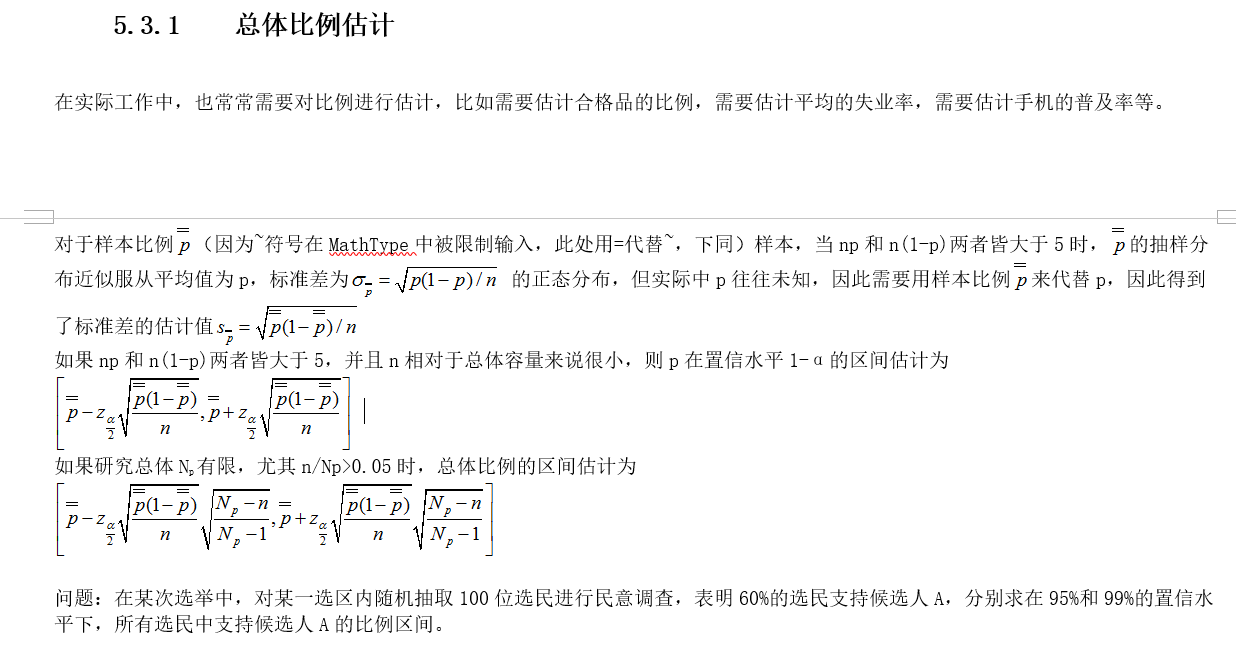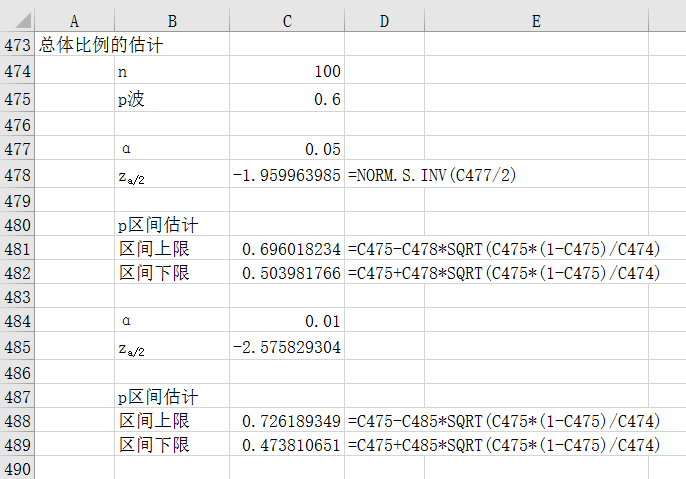展开全文• 但是，在实际应用中，类条件概率密度通常是未知的。那么，当先验概率和类条件概率密度都未知或者其中之一未知的情况下，该如何来进行类别判断呢？其实，只要我们能收集到一定数量的样本，根据统计学的知识，可以从...
1、概率密度函数
在分类器设计过程中（尤其是贝叶斯分类器），需要在类的先验概率和类条件概率密度均已知的情况下，按照一定的决策规则确定判别函数和决策面。但是，在实际应用中，类条件概率密度通常是未知的。那么，当先验概率和类条件概率密度都未知或者其中之一未知的情况下，该如何来进行类别判断呢？其实，只要我们能收集到一定数量的样本，根据统计学的知识，可以从样本集来推断总体概率分布。这种估计方法，通常称之为概率密度估计。它是机器学习的基本问题之一，其目的是根据训练样本来确定x（随机变量总体）的概率分布。密度估计分为参数估计和非参数估计两种。

2、参数估计
参数估计：根据对问题的一般性认识，假设随机变量服从某种分布（例如，正态分布），分布函数的参数可以通过训练数据来估计。参数估计可以分为监督参数估计和非监督参数估计两种。参数估计当中最常用的两种方法是最大似然估计法和贝叶斯估计法。

监督参数估计：样本所属类别及条件总体概率密度的形式已知，表征概率密度的某些参数是未知的。
非监督参数估计：已知样本所属的类别，但未知总体概率密度函数的形式，要求推断出概率密度本身。

3、非参数估计
非参数估计：已知样本所属的类别，但未知总体概率密度函数的形式，要求我们直接推断概率密度函数本身。即，不用模型，只利用训练数据本身来对概率密度做估计。
非参数估计常用的有直方图法和核方法两种；其中，核方法又分为Pazen窗法和KN近领法两种。展开全文• 最大似然估计与贝叶斯参数估计 前言 上一篇讲到了贝叶斯决策理论，其与贝叶斯估计是两种不同的思想。前者是根据先验概率P(ωi)P(ωi)P(\omega_i)和类条件概率密度p(x|ωi)p(x|ωi)p(x|\omega_i)来设计最优分类器...
最大似然估计与贝叶斯参数估计

前言
上一篇讲到了贝叶斯决策理论，其与贝叶斯估计是两种不同的思想。前者是根据先验概率P(ωi)P(ωi)<!--//--><![CDATA[//><!--
P(\omega_i)
//--><!]]>和类条件概率密度p(x|ωi)p(x|ωi)<!--//--><![CDATA[//><!--
p(x|\omega_i)
//--><!]]>来设计最优分类器。然而在实际应用中，通常得不到有关问题的概率结构的全部知识。我们只能利用手头的训练样本来估计问题中所涉及的先验概率和条件密度函数，并把这些估计的结果当作实际的先验概率和条件密度函数，然后再设计分类器。估计先验概率通常没有太大的困难，最大的困难在于估计类条件概率密度。其中主要的问题有两个:（1）在很多情况下，已有的训练样本数总是显得太少。（2）当用于表示特征的向量x的维数较大时，就会产生严重的计算复杂度问题。但是，如果我们事先已经知道参数的个数，并且先验知识允许我们能够把条件概率密度进行参数化，那么问题的难度就可以显著的降低。例如，我们可以正确的假设p(x|ωi)p(x|ωi)<!--//--><![CDATA[//><!--
p(x|\omega_i)
//--><!]]>是一个多元正态分布，其均值为μiμi<!--//--><![CDATA[//><!--
\mu_i
//--><!]]>,协方差矩阵为σiσi<!--//--><![CDATA[//><!--
\sigma_i
//--><!]]>（这两个参数的具体的值是未知的）。这样，我们就把问题从估计完全未知的概率密度p(x|ωi)p(x|ωi)<!--//--><![CDATA[//><!--
p(x|\omega_i)
//--><!]]>转化为估计参数μiμi<!--//--><![CDATA[//><!--
\mu_i
//--><!]]>和σiσi<!--//--><![CDATA[//><!--
\sigma_i
//--><!]]>。这种方法就称为参数估计。
2. 最大似然估计
最大似然估计把待估计的量看作是确定性的量，只是其取值未知。最佳估计就是使得产生已观测到的样本(即训练样本)的概率为最大的那个值。基本原理如下图所示：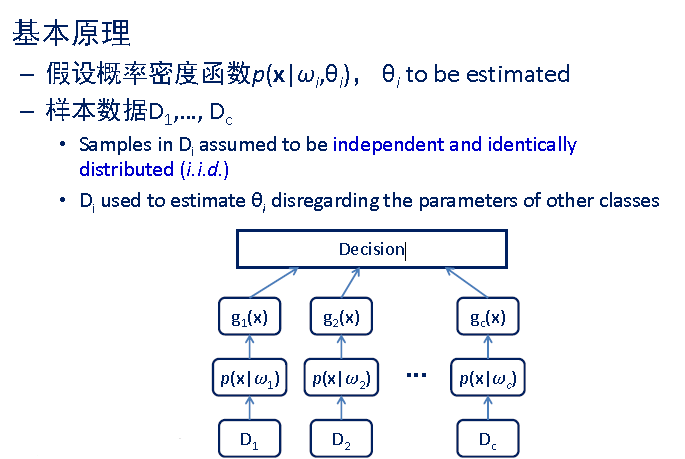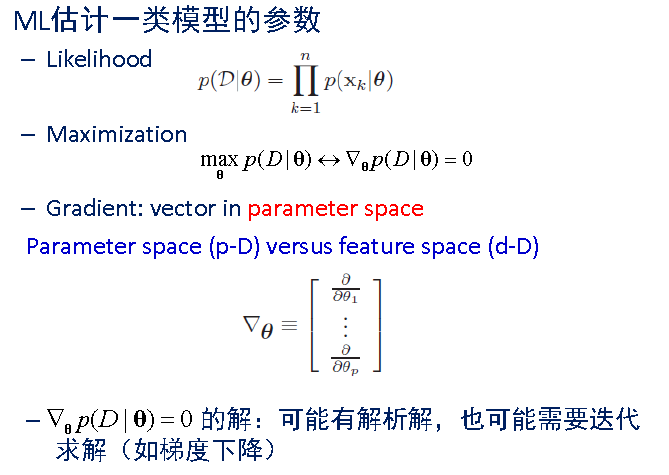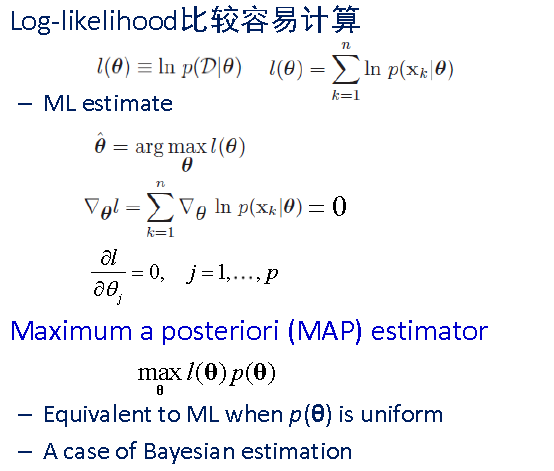贝叶斯估计
与最大似然估计不同，贝叶斯估计把待估计的参数看成是符合某种先验概率分布的随机变量。对样本进行观测的过程，就是把先验概率密度转化为后验概率密度，这样就利用样本的信息修正了对参数的初始估计值。在贝叶斯估计中，一个典型的效果就是，每得到新的观测样本，都使得后验概率密度函数变得更加尖锐，使其在待估参数的真实值附近形成最大的尖峰，这个现象就称为”贝叶斯”学习过程。无论使用何种参数估计方法，在参数估计完成后，我们都使用后验概率作为分类准则。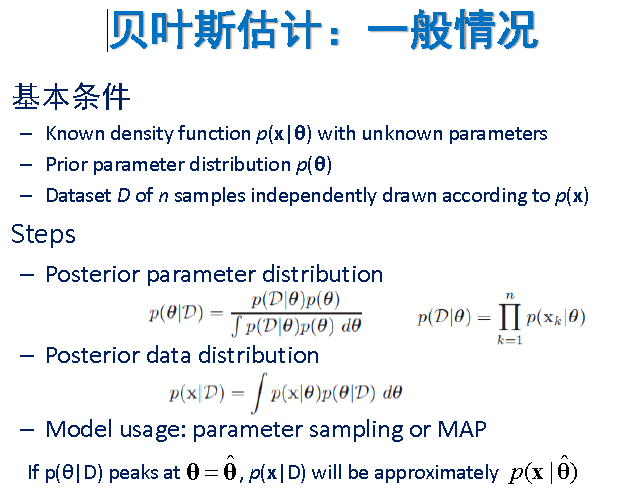另外这篇博客http://blog.csdn.net/u011508640/article/details/72815981#comments个人感觉讲的也很到位。
展开全文• 统计推断包括参数估计和假设检验。参数估计就是用样本指标（统计量）来估计总体指标（参数）。 一、参数估计基础-Z分布 在统计应用中，可以把任何一个均数为，标准差为的正态分布转变为,的标准正态分布，即将...统计推断 区间估计
• “除了需要对单个总体的均值进行估计，在实际工作中，有时需要对来自两个总体的均值之差进行估计，如需要判断两户居民每月用电平均相差多少，需要计算两支股票收益率的平均差别等。” 解答：
• 我们是利用先验概率 P(c)P(c)P(c) 和 类条件概率密度 p(x∣c)p(x|c)p(x∣c) 来设计最优分类器，但是在实际应用中，通常是拿不到概率结构的全部知识的，因此我们就需要利用这些训练样本来估计问题中涉及的先验概率和...
• 针对复杂的电磁环境对跳频信号检测所产生的影响，提出了一种新的基于图像处理的跳频信号参数估计方法。首先将短时傅里叶变换（STFT）后的时频图处理为二维图像，然后根据电磁环境中噪声在时频图中的表现形式，对时频...软件
• 但是，在实际的工程应用中，大多数情况我们是不知道类的条件概率密度函数的，甚至不知道该函数的形式，那么参数估计也就无从谈起了。因此，需要直接从样本数据出发，得到类条件概率密度函数。   一种很自然的想法...
• 仿真结果表明，基于改进的物种形成粒子群算法能够搜索到与跳频信号分量相匹配的原子，与平滑伪魏格纳分布相比，提出的参数估计算法在低信噪比下具有较小的估计方差，更加适宜于电子战的实际应用跳频信号
• 较为明确、发展相当成熟的研究领域, 在思想方法、理论基础、实际应用等诸多方面都有丰富的研究成果. 进入新世纪, 伴随着 科学技术的突飞猛进, 新学科、新研究领域不断涌现, 给传统的系统辨识带来了新的挑战与机遇. ...
• 我们已经知道了如何根据先验概率P(w1)和类条件概率密度p(x|wi)来设计分类器，但实际应用中通常得不到有关问题的概率结构的全部知识，只有一些模糊而笼统的先验知识和训练样本。这时我们利用训练样本来估计问题中设计...机器学习 人工智能 最大似然
• 负二项回归模型下双参数估计的有效性研究，姚程，黎雅莲，在实际应用中分析计数数据时经常会用到负二项分布（NB）回归模型这一典型广义线性模型。复共线性问题的提出使得经典的极大似然估�
• 本文以已实现核估计作为波动率非参数估计的代表，构建了一种能自动从实际数据中确定最优窗宽的算法.理论分析的结果表明：算法具有稳定性，其所确定的窗宽是最优窗宽的无偏一致估计量，收敛速度为O（n-1/5）.实际数据...
• 但是，在实际的工程应用中，类概率密度函数往往是未可知的。即使把类概率密度函数近似为正态分布函数，其分布的均值和方差也是未知的。 因此，我们需要从已知的有限的样本中，尽可能地估计出类条件概率密度函数的...
• 本文在前人工作的基础上，结合通信侦察和干扰的实际应用要求，主要讨论了常 见数字通信信号的调制参数估计和DS、混合SFH/DS扩频信号的扩频参数估计问题 以及针对FSK和PSK的干扰样式研究。所做的工作主要包括： 1、...
• 7、结合信号侦察的实际应用，讨论了数字通信信号子类分离的算法。提出了基 于判决树结构与综合考虑所有分类特征的常见数字通信信号的自动分类算法。将支持 矢量机分类器推广到信号子类的分离，使得构造的分类器对新...
• 参数估计的无偏性、有效性以及一致性 无偏性 定义式： 无偏估计是用样本统计量来估计总体参数时的一种无偏推断。估计量的数学期望等于被估计参数的真实值，则称此此估计量为被估计参数的无偏估计，即具有无偏性，是...
• 论文研究-时变弹性系数生产函数的非参数估计.pdf, 提出了时变弹性系数生产函数模型,该模型刻画了弹性系数不再是常数而是随时间变化而变化的函数,并且去除了古典生产函数...
• 实际生产的产品，其长度X假定服从正态分布N(μ,σ2),σ2N(\mu, \sigma^2), \sigma^2N(μ,σ2),σ2未知，现从该厂生产的一批产品中抽取6件，得尺寸数据如下： 32.56， 29.66， 31.64， 30.00， 31.87， 31.87， 31.03...机器学习
• 显然，当假设模型与实际非常接近的情况下，参数化方法相对于非参数化方法能提供更为精确的谱估计值；但是，在研究信号的信息极少甚至没有的应用中，功率谱密度估计的非参数化方法仍然有用。 谱估计参数化...
• 无偏性 定义式： E(θ^)=θ 无偏估计是用样本统计量来估计总体参数时的一种无偏推断...无偏估计常被应用于测验分数统计中。 无偏性的实际意义是指没有系统性的偏差。统计推断的误差有系统误差和随机误差两种。无论...
• 1.GLM参数估计——极大似然法 ...事实上，实际应用中使用最多的分布就是指数分布族，所以这样的简化可以节省很多理论上的冗长论述，也不会限制实际应用。 如前文如述，指数分布族的概率密度函
• 在介绍正交频分复用（OFDM）技术基本原理和基本接收模型的基础上，提出将空间谱估计中的多重信号分类（MUSIC）算法应用到OFDM系统参数估计中。经过MATLAB实验仿真表明，在没有信号先验知识的情况下，此算法对...
• 结果表明,采用矩阵束法可以较精确地估计出频率依赖性UWB信道参数,而且比扩展Prony法的估计误差要小得多,具有更高的抗噪性能。最后,还结合一个实际的井下频率依赖性UWB信道,利用矩阵束算法进行了信道重现。结果与原...
• 为此，提出一种基于地理特征提取与非参数核密度估计的空间负荷分布规律研究方法。按某地的实际用地生成功能小区，提取功能小区的地理特征信息，并给定其用地类型；然后根据提取的信息，应用聚类算法对功能小区分类；...
• 基于研究卡尔曼滤波算法在锂电池荷电状态估计和监测中应用效果的目的，本文通过建立Thevenin电池模型，结合锂电池恒定电流充放电实验数据，有效模拟出电池实际工作特性，并分别采用传统卡尔曼滤波（KF）和扩展卡尔曼...
• （一）单个正态总体N(μ,σ2)N(\mu,\sigma^2)N(μ,σ2)的区间估计 均值μ\muμ的置信区间 (1) σ2\sigma^2σ2已知 设X1,...,XnX_1,...,X_nX1​,...,Xn​是取自N(μ,σ2)N(\mu,\sigma^2)N(μ,σ2)的样本，σ2\sigma...机器学习...